Our World Statistics Day conversations have been a great reminder of how much statistics can inform our lives. Do you have an example of how statistics has made a difference in your life? Share your story with the Community!
Choose Language Hide Translation Bar

At a recent Building Better Models seminar, someone asked me, “If you have a factor that has a curved relationship with a response, can a decision tree model be used to model that relationship?” To show that this is indeed possible, I created a simple simulated data set with 200 rows, based on the model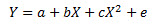where a, b, c are constants, and e is a normally distributed random variable. A plot of the data, along with the fitted quadratic regression model, is shown in Figure 1.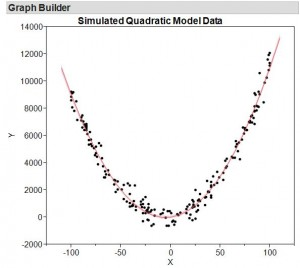So what does a decision tree model look like when fitted to this data? To find out, I used the Partition platform (Analyze > Modeling > Partition) to fit a simple decision tree. A compact view of the decision tree model is in Figure 2.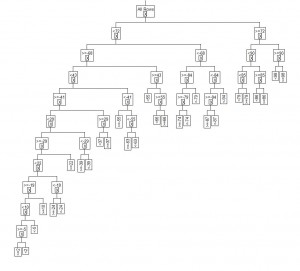Examining a plot of the fitted model (Figure 3), we see that the general quadratic model shape is captured by the decision tree model. Inside the range of the X data (-100 < X < 100), this model makes predictions that are very similar to the quadratic model. In fact, both models have the same average prediction errors, and the R2 for both models is 0.98 . This is nice because decision trees make no assumption about the shape of the relationship between the Y and the X.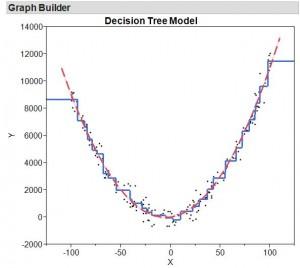It is important to know that the decision tree model fit here was the largest/most complex model that was possible, with the only restriction that each terminal node in the tree was required to have a minimum of five data points. The noise in this data is relatively small compared to the overall variability in this data, which explains why both the quadratic and partition models can have such a good fit.

But, what if we have much noisier data? Consider a second simulated data set from the same quadratic model, but where the standard deviation of the random error is 10 times larger. You can see the fitted quadratic model and the full decision tree model overlaid with the data in Figure 4.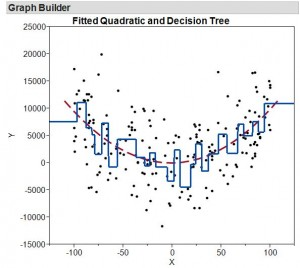In this case, the decision tree no longer looks like it is capturing the quadratic nature of the data well. This is because the partition model is overfit. Too much of the random error present in the data is assigned to the model, which results in a model that is too complex.

To build a better decision tree model that will capture the essential nature of the data without overfitting, we will use a random holdout of 20% of the data as a validation set to help automatically choose the size of the decision tree model. This is a feature built into JMP Pro, the advanced analytics version of JMP. The resulting model was chosen based on the validation set and only has five terminal nodes. The overlay of this simpler decision tree model (Figure 5) shows that the decision tree is now capturing that overall quadratic nature of the data. So, the answer to the original question is still “yes,” and JMP Pro helps you to do it in a fast , automatic fashion.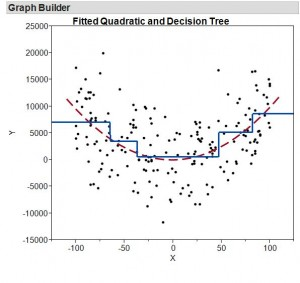Article Labels

There are no labels assigned to this post.

Article Tags
1 Comment
Visitor

Eric Cai wrote:

Hi Sam,

Thanks for this very clear and informative post. Could you please explain why you used holdout validation instead of cross-validation? Isn't holdout validation prone to variation from which data were held out as the validation set? Cross-validation can overcome this variation, and this is the validation method that I usually use. I would be glad to learn about the advantages of holdout validation.